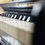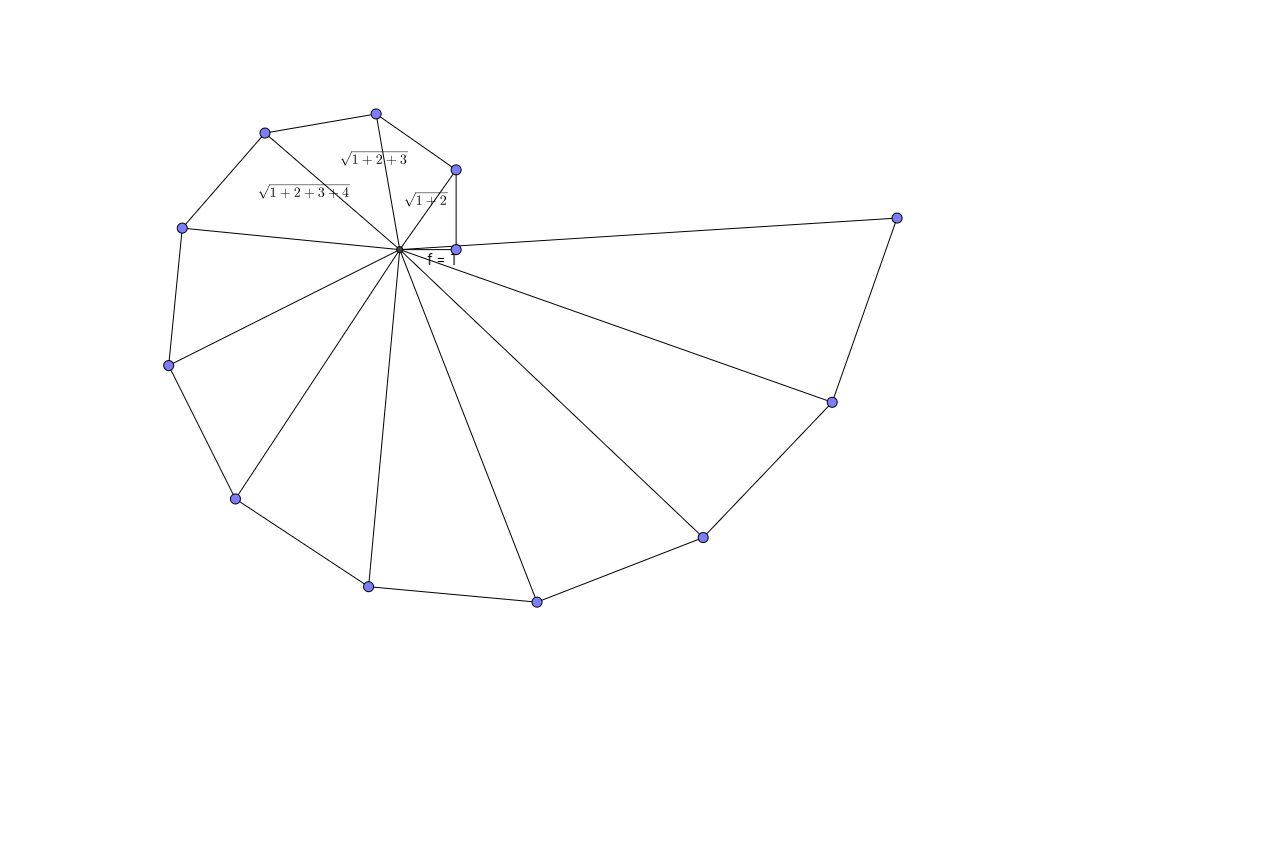# Proof

Show that, for any positive integer $n\geq 1$ it is true that: $\displaystyle \sum_{k=1}^{n} \sqrt{k} \geq \sqrt\sum_{k=1}^{n} k}$Note by Hjalmar Orellana Soto
4 years, 7 months ago

This discussion board is a place to discuss our Daily Challenges and the math and science related to those challenges. Explanations are more than just a solution — they should explain the steps and thinking strategies that you used to obtain the solution. Comments should further the discussion of math and science.

When posting on Brilliant:

• Use the emojis to react to an explanation, whether you're congratulating a job well done , or just really confused .
• Ask specific questions about the challenge or the steps in somebody's explanation. Well-posed questions can add a lot to the discussion, but posting "I don't understand!" doesn't help anyone.
• Try to contribute something new to the discussion, whether it is an extension, generalization or other idea related to the challenge.

MarkdownAppears as
*italics* or _italics_ italics
**bold** or __bold__ bold
- bulleted- list
• bulleted
• list
1. numbered2. list
1. numbered
2. list
Note: you must add a full line of space before and after lists for them to show up correctly
paragraph 1paragraph 2

paragraph 1

paragraph 2

[example link](https://brilliant.org)example link
> This is a quote
This is a quote
    # I indented these lines
# 4 spaces, and now they show
# up as a code block.

print "hello world"
# I indented these lines
# 4 spaces, and now they show
# up as a code block.

print "hello world"
MathAppears as
Remember to wrap math in $$ ... $$ or $ ... $ to ensure proper formatting.
2 \times 3 $2 \times 3$
2^{34} $2^{34}$
a_{i-1} $a_{i-1}$
\frac{2}{3} $\frac{2}{3}$
\sqrt{2} $\sqrt{2}$
\sum_{i=1}^3 $\sum_{i=1}^3$
\sin \theta $\sin \theta$
\boxed{123} $\boxed{123}$

Sort by:

for $n\geq 2$ it can be easily proven by applying triangle inequality recursively

assume a,b,c are three terms in the seuence

we have $\sqrt{a}+\sqrt{b}\geq \sqrt{a+b}$ as $\sqrt{a},\sqrt{b},\sqrt{a+b}$ form sides of a right triangle

now we have $\sqrt{a}+\sqrt{b}+\sqrt{c}\geq \sqrt{a+b}+\sqrt{c}\geq\sqrt{a+b+c}$ and so on....sum of each of the outer sides of the spiral form the LHS of the equation,and RHS is given by the line to centre

also note that for n=1 the line to centre is same as side, the only point of equality

- 4 years, 6 months ago

very nice solution, Anirudh

- 4 years, 6 months ago

thank you :)

- 4 years, 6 months ago

$LHS^2 = 1+2+\cdots+k + 2\sum_{1\le x and equallity occurs when $n=1$

- 4 years, 6 months ago

We want to prove that $\sqrt{1} + \sqrt{2} + \dots + \sqrt{n} \ge \sqrt{1 + 2 + \dots + n}.$ Squaring the left-hand side, we get a term of $\sqrt{k} \cdot \sqrt{k} = k$ for each $1 \le k \le n$. The inequality follows.

- 4 years, 6 months ago

In addition to the problem.... Is this necessary information for saying that the limit below converges? $\lim_{x\to \infty} \dfrac\sqrt{\sum_{n=1}^{x}n}}\sum_{n=1}^{x}\sqrt{n}$

- 4 years, 6 months ago

(Assuming I'm interpreting you correctly,) the essence of what you're asking here is:

If $a_n, b_n$ are sequences of (positive) reals, such that $a_n \geq b_n$, does $\lim \frac{ b_n } { a_n }$ exist?
If yes, why?
If no, what is a counter example? What other assumptions do we need to add to guarantee a true statement?

Staff - 4 years, 6 months ago

Exactly that is what I ask, I was thinking of asking for the value of $\displaystyle \sum_{n=1}^{\infty} (\frac{\sqrt{\sum_{k=1}^{n}n}}{\sum_{k=1}^{n}\sqrt{n}})$ but I really don't know how to approach it

- 4 years, 6 months ago

The answer to "does $\lim \frac{ b_n } { a_n }$ exist?" is no for general sequences. Do you see an obvious counter example?

For your recent comment, are you looking for the summation, or for the limit of the term (as in the prior comment)?

Staff - 4 years, 6 months ago

The answer to the limit question is yes for this case, using the same argument which is used for $\lim_{x\to \infty} \frac{x}{e^x}$.... and I'm looking for the summation

- 4 years, 6 months ago

See How to ask for help to understand how to provide proper context of what you want. E.g. You can see that people quickly established the inequality, but that doesn't help with your concern.

Staff - 4 years, 6 months ago

The summation is something else, something I supposed and now I'm trying to approach, and I think the inequality may help for knowing if the summation converges... and then I'll try to approach the value of the summation, I don't know if I'm explaining right...

- 4 years, 6 months ago

Asymptotically, $\sqrt{1} + \sqrt{2} + \dots + \sqrt{n}$ can be approximated by the integral $\int_0^n \sqrt{x} \ dx = \frac{2}{3} n^{3/2}.$ And of course, $\sqrt{1 + 2 + \dots + n} = \sqrt{\frac{n(n + 1)}{2}}.$ That should help answer your question.

- 4 years, 6 months ago